# Volume

Conjugate variables
of thermodynamics
Pressure Volume
Temperature Entropy
Chem. potential Particle no.

The amount of space occupied by an object or system is called the volume of the object or system. The volume of an object is one of the physical properties of the object. (For other meanings of the term, see Additional meanings of "volume" below.)

The volume of a solid object is given a numerical value that quantifies the amount of three-dimensional space it occupies. A one-dimensional object, such as a line in mathematics, or a two-dimensional object, such as a square, is assigned zero volume in three-dimensional space. In the thermodynamics of non-viscous fluids, volume is regarded as a "conjugate variable" to pressure. If pressure on the fluid is increased, its volume decreases; conversely, if pressure on the fluid is decreased, its volume increases.

Volume is sometimes distinguished from the capacity of a container. The term capacity is used to indicate how much a container can hold (commonly measured in liters or its derived units), and volume indicates how much space the object displaces (commonly measured in cubic meters or its derived units). Alternatively, in a capacity management setting, capacity is defined as volume over a specified time period.

## Volume formulae

Common equations for volume:
Shape Equation Variables
A cube: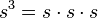$s^3 = s \cdot s \cdot s$ s = length of a side
A rectangular prism:$l \cdot w \cdot h$ l = length, w = width, h = height
A cylinder (circular prism):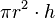$\pi r^2 \cdot h$ r = radius of circular face, h = distance between faces
Any prism that has a constant cross sectional area along the height: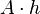$A \cdot h$ A = area of the base, h = height
A sphere: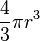$\frac{4}{3} \pi r^3$ r = radius of sphere
which is the first integral of the formula for Surface Area of a sphere
An ellipsoid: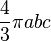$\frac{4}{3} \pi abc$ a, b, c = semi-axes of ellipsoid
A pyramid: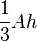$\frac{1}{3} A h$ A = area of base, h = height from base to apex
A cone (circular-based pyramid):$\frac{1}{3} \pi r^2 h$ r = radius of circle at base, h = distance from base to tip
Any figure (integral calculus required)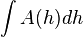$\int A(h) dh$ h = any dimension of the figure, A(h) = area of the cross-sections perpendicular to h described as a function of the position along h.
(This will work for any figure, no matter if the prism is slanted or the cross-sections change shape).

Mathematically, the volume of a body may be defined by means of integral calculus. In this approach, the volume of the body is taken to be approximately equal to the sum of volumes of a large number of small cubes or concentric cylindrical shells, and adding the individual volumes of those shapes.

## Volume measures: United States

U.S. customary units of volume include the following:

• U.S. fluid ounce, about 29.6 milliliters (mL)
• U.S. liquid pint = 16 fluid ounces, or about 473 mL
• U.S. dry pint = 1/64 U.S. bushel, or about 551 mL (used for things such as blueberries)
• U.S. liquid quart = 32 fluid ounces (two U.S. pints), or about 946 mL
• U.S. dry quart = 1/32 U.S. bushel, or about 1.101 liters (L)
• U.S. liquid gallon = 128 fluid ounces or four U.S. quarts, about 3.785 L
• U.S. dry gallon = 1/8 U.S. bushel, or about 4.405 L
• U.S. (dry level) bushel = 2150.42 cubic inches, or about 35.239 L
• cubic inch = 16.387 064 centimeters3
• cubic foot = 1,728 inches3 ≈ 28.317 decimeters3
• cubic yard = 27 feet3 ≈ 0.7646 meters3
• cubic mile = 5,451,776,000 yards3 = 3,379,200 acre-feet ≈ 4.168 kilometers3

The acre foot is often used in measuring the volume of water in a reservoir or aquifer. It is the volume of water that would cover an area of one acre to a depth of one foot. It is equivalent to 43,560 cubic feet or 1233.481 cubic meters.

## Volume measures: UK

The United Kingdom is increasingly using units of volume according to the SI metric system, namely, the cubic meter and liter. However, some former units of volume are still being used in varying degrees.

Imperial units of volume:

• UK fluid ounce = about 28.4 mL (this equals the volume of an avoirdupois ounce of water under certain conditions)
• UK pint = 20 fluid ounces, or about 568 mL
• UK quart = 40 ounces or two pints, 1.137 L
• UK gallon = 4 quarts, or exactly 4.546 09 L

## Volume measures: cooking

Traditional cooking measures for volume also include:

• teaspoon = 1/6 U.S. fluid ounce (about 4.929 mL)
• teaspoon = 5 mL (metric)
• tablespoon = ½ U.S. fluid ounce or 3 teaspoons (about 14.79 mL)
• tablespoon = ½ Imperial fluid ounce or 3 teaspoons (about 14.21 mL) (Canada)
• tablespoon = 15 mL or 3 teaspoons (metric)
• tablespoon = 5 fluidrams (about 17.76 mL) (British)
• cup = 8 U.S. fluid ounces or one-half U.S. liquid pint (about 237 mL)
• cup = 8 Imperial fluid ounces or one-half fluid pint (about 227 mL) (Canada)
• cup = 250 mL (metric)

## Relationship to density

The volume of an object is equal to its mass divided by its average density (the term "average density" is used for an object that does not have uniform density). This is a rearrangement of the calculation of density as mass per unit volume.

The term "specific volume" is used for volume divided by mass, expressed in units such as cubic meters per kilogram (m³•kg-1). It is the reciprocal of density.

## Orders of magnitude (volume)

List of orders of magnitude for volume
Factor (meters³*) Multiple Value
10−105 4×10−105 m3 is the Planck volume
10−45 Volume of a proton
10−33 Volume of a hydrogen atom (6.54×10-32 meters3)
10−21 1 attoliter Volume of a typical virus (5 attoliters)
10−15 1 picoliter A small grain of sand (0.063 millimeter diameter, 3 micrograms, 130 picoliters)
10−12 1 nanoliter A medium grain of sand (0.5 millimeter diameter, 1.5 milligrams, 62 nanoliters)
10−9 1 microliter A large grain of sand (2.0 millimeter diameter, 95 milligrams, 4 microliters)
10−6 1 milliliter
(1 cubic centimeter)
1 teaspoon = 3.55 mL to 5 mL

1 tablespoon = 14.2 mL to 20 mL

10−3 1 liter
(1 cubic decimeter)
1 U.S. quart = 0.95 liters;
1 United Kingdom quart = 1.14 liters
100 1000 liters Fuel tank for a 12-passenger turboprop airplane
103 1000 cubic meters
(1 million liters)
A medium-size forest pond.
An Olympic-size swimming pool, 25 meters by 50 meters by 2 meters deep, holds at least 2.5 megaliters.
106 1 million cubic meters
109 1 cubic kilometer (km3) Volume of Lake Mead (Hoover Dam) = 35.2 km3

Volume of crude oil on Earth = ~300 km3

1012 1000 cubic kilometers Volume of Lake Superior = 12,232 km3
1015
1018 Volume of water in all Earth oceans = 1.3×1018
1021 Volume of Earth = ~1×1021 m3
1024 Volume of Jupiter = ~1×1025 m3
1027 Volume of the Sun = ~1×1027 m3
1030 Volume of a red giant the same mass as the Sun = ~5×1032 m3
1033 Volume of Betelgeuse = ~2.75×1035 m3
1054 Volume of small dwarf galaxy like NGC 1705 = ~3×1055 m3
1057 Volume of dwarf galaxy like the Large Magellanic Cloud = ~3×1058 m3
1060 Volume of galaxy like the Milky Way = ~3.3×1061 m3
1066 Volume of the Local Group (galaxy group that includes the Milky Way) = ~5×1068 m3
1072 Volume of the Virgo Supercluster = ~4×1073 m3
1081 Approximate volume of the observable universe 1.6×1081 m3